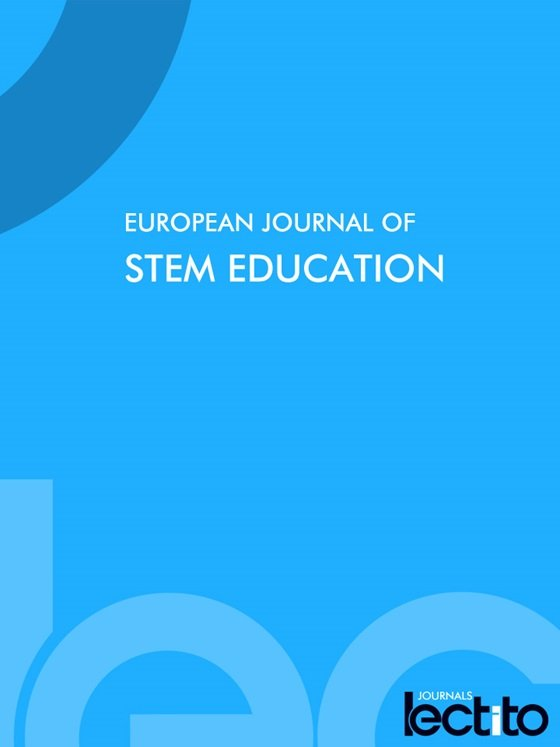Research Article
2021, 6(1), Article No: 09

## Promoting Geometric Reasoning through Artistic Constructions

Published in Volume 6 Issue 1: 09 Nov 2021
View: 2153

# Abstract

In order to promote geometric understanding, teachers frequently use hands-on activities. Such activities can be used to expound upon the declarative statements and theorems of geometry. Using a compass, straightedge, and protractor, students are able to actively build conceptions involving bisectors, midpoints, and perpendicular lines. Additionally, activities that require students to problem-solve and formulate problems, using their construction knowledge and skills, can reinforce and strengthen that which they have learned. This article describes STEAM instruction with high school geometry students designed to productively integrate geometric constructions, digital technology, elements of art, and principles of design to enhance students’ geometric reasoning.

# INTRODUCTION

Geometry and spatial sense provide students with understandings and ways of thinking that can be applied in a variety of contexts. In addition, geometric reasoning offers ways to interpret, describe, and reflect on our physical environment and can serve as a tool for study in other areas of mathematics, the sciences, and various real-world situations (National Council for Teachers of Mathematics, 2000, p. 41). In the United States, Geometry not only frequently represents a high school student’s first formal introduction to abstract reasoning, but the Common Core’s Geometry conceptual category also places “new emphasis on geometry proof and construction (prove geometric theorems, make geometric constructions)” (Harel, 2014, p. 25)—concepts well established as one of the most difficult for students and their teachers (e.g., Battista and Clements, 1992; Chavula and Nkhata, 2019; Erduran and Yesildere, 2010; Harel and Sowder, 2007; Hart, 1994; Yackel and Hanna, 2003).

Prior research has examined instruction designed to promote students’ mathematical reasoning, particularly as it relates to proof, through a focus on increasing teachers’ awareness of students’ cognitive skills, attitudes, and misconceptions (Mistretta, 2000), students’ intellectual need (Harel, 2013; 2014), technology (e.g., Battista, 1998, Hollebrands, 2007), and the organization of students’ knowledge (Lawson and Chinnappan, 2000). In this article, we describe a high school Geometry teacher’s attempts to promote a STEAM environment by providing her students with opportunities to use geometric constructions, and mathematically challenging and aesthetically pleasing geometric figures in mathematical proofs; specifically, the report addresses the following research question: How can a high school Geometry class integrate geometric constructions, digital technology, elements of art, and principles of design to enhance students’ geometric reasoning?

# GEOMETRIC PROOFS AND CONSTRUCTIONS

In Geometry, proof can take on different forms, such as: two-column, informal, indirect, and paragraph proofs. Geometric constructions are valuable to proof because of the hands-on way that students are motivated to visualize theorems as they “come to life” with compass and straightedge. According to Sanders (1998), “Geometric constructions can enrich students’ visualization and comprehension of geometry, lay a foundation for analysis and deductive proof, provide opportunities for teachers to address multiple intelligences, and allow students to apply their creativity to mathematics” (p. 554). Integrating hands-on activities and proof through geometric constructions, not only promotes greater student interest, but also provides students with more meaningful learning experiences.

Research by Middleton (1995) indicates that hands-on activities are considered to be motivational by both teachers and students. Furthermore, Bergin (1999) describes hands-on activities to be one of the situational factors that positively influences classroom (i.e., student) interest. According to Bergin (1999), “People seem to be interested in hands-on activities, activities in which they manipulate materials, move around, and engage learning in a physical way” (p. 92). In her own classroom, the second author engages students in such activities that include straightedge and compass constructions. Furthermore, the second author regularly takes a practical approach to STEAM education through arts integration, which Liao (2019) asserts is often “discussed at the level of instructional approach and lessons, although its larger goal is also ‘integration,’ which can be implemented in a variety of ways” (p. 41).

# STEAM EDUCATION

Goldsmith et al. (2016) suggest the “development of visual-spatial thinking through the visual arts could support geometry learning for students who are not succeeding in mathematics classes” (p. 56). In addition to providing much-needed motivation, an important value of explicitly connecting mathematics and art is that it “can illuminate pupils’ understanding[s] of some of its purpose” (Hickman and Huckstep, 2003, p. 2). The mathematics community often talks about beautiful or aesthetically pleasing theorems or theorem proofs in much the same way the art community talks about beauty (Malkevitch, 2003, Introduction section, para. 4). For Hickman and Huckstep (2003) there is “an undeniably aesthetic dimension to mathematics . . . [that] is not simply confined to the notion of an ‘elegant solution’ to a problem” (p. 4). Rather, mathematics itself has “aesthetic properties and . . . one can have an aesthetic experience through mathematics, while acknowledging that aesthetics is not confined to artistic activities” (Hickman and Huckstep, 2003, p. 4).

STEAM education has been described as “intentionally integrating the concepts and practices articulated with 21st-century skills in curriculum, instruction, assessment, and enrichment, while purposefully integrating science, technology, engineering, arts (including but not limited to the visual and performing arts), and mathematics” (Gettings, 2016, p. 10). The activities described here incorporate STEAM concepts to promote creativity, digital technology (i.e., dynamic geometry software), and Thuneberg et al.’s (2018) assertion that the “aesthetic elements of . . . art promote understanding of mathematical concepts by exposing students to concrete space and shape experiences” (p. 153). In the following sections, we describe the second author’s implementation of a sequence of high school mathematics activities designed to provide students with opportunities to engage in geometric reasoning and develop meaningful understandings involving geometry and proof through artistic compass and straightedge constructions and digital technology. Taking note of Gettings’ (2016) warning regarding the danger of superficially including art in STEM projects, the sequence of activities utilize art to enhance students’ geometric reasoning.

# METHODS

The activities described below are utilized by the second author as part of her Geometry curriculum at a small private suburban high school in the midwestern United States. Although the school is a private, college-preparatory institution, 40% of the student population receive financial aid, and 25% of students come from minority populations (below the state average of 30.4%). Furthermore, the average class size of 19 students is smaller than the state average of 20.7 students.

Data is comprised of class handouts (e.g., activity sheets); student work (written and using GeoGebra); the second author’s recollections of her students’ questions, discussions, assertions, and reactions to the activities; and video recordings of discussions between both authors regarding the rationale for each activity and their sequencing, anticipated and actual student responses, and potential lesson modifications.

Throughout the sequence of activities, the second author and her students investigate (as a class) segment and angle constructions, and angle and segment congruence. The first half of the four-day lesson (comprised of four 45-minute class periods) culminates with students individually constructing a perpendicular bisector to a given line segment. The Common Core content standard addressed in these activities are illustrated in Table 1.

Table 1. Common Core Content Standard Addressed by Lesson (NGA Center & CCSSO, 2010, p. 76)

 Conceptual Category Geometry (G) Domain Congruence (CO) Cluster D. Make geometric constructions Standard 12. Make formal geometric constructions with a variety of tools and methods (compass and straightedge, string, reflective devices, paper folding, dynamic geometric software, etc.). Copying a segment; copying an angle; bisecting a segment; bisecting an angle; constructing perpendicular lines, including the perpendicular bisector of a line segment; and constructing a line parallel to a given line through a point not on the line.

Proof as an interactive, class activity, requires that students create logical arguments by employing meanings and reasoning, explicating their own thinking, and critiquing the reasoning of others. As such, the activities provide students with opportunities to engage in several of the Common Core Standards for Mathematical Practice (frequently identified as MPs). In particular, students engage in MP3 (Construct viable arguments and critique the reasoning of others) by making conjectures and building a logical progression of statements to explore the truth of their conjectures, by justifying their conclusions, communicating them to others, and responding to the arguments of their classmates and teacher (NGA Center & CCSSO, 2010, pp. 6-7). In addition, students will need to attend to precision (MP6), by examining claims and making explicit use of definitions (NGA Center & CCSSO, 2010, p. 7), and use appropriate tools strategically (MP5) by becoming familiar with and utilizing tools (i.e., compass and straightedge) to “explore and deepen their understanding of concepts” (NGA Center & CCSSO, 2010, p. 7). Along with the mathematical content and practice standards described above, the activities also address two state high school visual arts standards (Ohio Department of Education, 2020, p. 4): “Integrate selected elements of art and principles of design to construct works of art” and “Increase relevant vocabulary to describe and analyze components related to visual art.” Finally, we employ a definition for geometric reasoning as provided in NCTM’s (2000) geometry standard, as to: “analyze characteristics and properties of two- and three- dimensional geometric shapes and develop mathematical arguments about geometric relationships; apply transformations and use symmetry to analyze mathematical situations; and use visualization, spatial reasoning, and geometric modeling to solve problems” (p. 41).

# ACTIVITY HIGHLIGHTS

In order to support students in using their compass and straightedge, the second author projects her sample constructions directly to a SMART Board. The class completes most of the requested constructions together, but students are occasionally asked to solve problems on their own so they can internally develop the construction using their geometric tools. By using this more moderate pace throughout the activities, the second author provides time for students to create their own relational system for constructions (van Hiele, 1959/1985; van Hiele and van Hiele-Geldof, 1958).

Some of the constructions involving line segments that students are requested to complete are displayed in Figure 1. Note that students’ constructions are completed on a separate sheet of paper and completion of these constructions conclude the first 45-minute class period.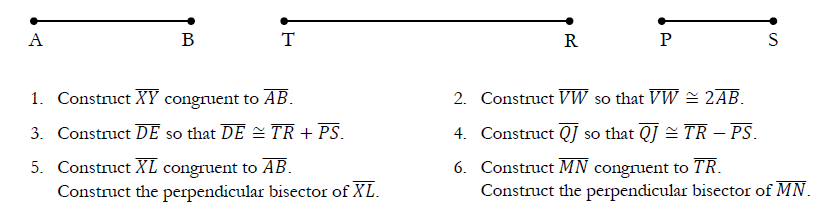Figure 1. Line Segment Constructions

Throughout the line segment construction portion of the lesson, students are motivated to share their thinking and reasoning both in pairs and as a whole class. When necessary, students are prompted to use appropriate terminology (e.g., congruent). According to the second author, students typically extend the activity by asking one another questions such as:

• “Describe how to construct a perpendicular bisector of segment TR that is congruent to segment AB”; that is, describe how to construct a perpendicular bisector of $$\overline{\text{TR}}$$ that is congruent to $$\overline{\text{AB}}$$.

• “Describe how to construct a perpendicular bisector of segment AB that is congruent to twice the length of segment PS”; that is, describe how to construct a perpendicular bisector of $$\overline{\text{AB}}$$ that is congruent to 2$$\overline{\text{PS}}$$.

• “Describe how to construct a perpendicular bisector of a segment with length equal to the sum of PS and TR that is congruent to 3 times the length of segment AB”; that is, describe how to construct a perpendicular bisector of $$\overline{\text{PS}} + \overline{\text{TR}}$$ that is congruent to 3$$\overline{\text{AB}}$$.

These examples show students coordinating their understandings for congruence and perpendicular bisector and visualizing geometric constructions to formulate problems. A long line of research has shown the potential for problem posing to benefit student learning in mathematics (e.g., Cai et al., 2013; English, 1998; Yuan and Sriraman, 2011). The second author always allows time for students to complete some of these student-formulated problems.

Students also frequently question—either themselves, one another, or their teacher (i.e., second author)—whether attempts to construct $$\overline{\text{PS}} - \overline{\text{AB}}$$ could support their understanding of what it means for the “subtraction of a larger number from a smaller number to yield a negative result.” As with students’ other extension questions, the second author provides students with time to address this question prior to moving on to angle constructions. The second author asserts she must handle such instances with care and focus students’ attention on the definition of the length of a line segment as “the distance between its endpoints” and that, as a distance, this length cannot be negative. Therefore, the line segment $$\overline{\text{PS}} - \overline{\text{AB}}$$ does not exist and the second author promotes (to her students) an understanding that the “difference of two line segments” is not the same as arithmetic subtraction.

Discussions between the two authors have included adding the question: “Describe how to construct $$\overline{\text{FG}}$$ so that $$4\overline{\text{FG}} \cong \overline{\text{TR}}$$ (or $$\overline{\text{FG}} \cong \frac{1}{4}\overline{\text{TR}}$$).” We concluded that in future classes, the second author would ask half of her students (working in pairs) to construct $$\overline{\text{FG}}$$ so that $$4\overline{\text{FG}} \cong \overline{\text{TR}}$$ and the other half of students (also working in pairs) to construct $$\overline{\text{FG}}$$ so that $$\overline{\text{FG}} \cong \frac{1}{4}\overline{\text{TR}}$$. Once these constructions are completed, the whole class would be asked to compare these two constructions in terms of their “ease of construction” and similarity of results.

Some of the angle constructions that students are requested to complete are illustrated in Figure 2. Note again that students’ constructions are completed on a separate sheet of paper and these constructions comprise part of the second 45-minute class period.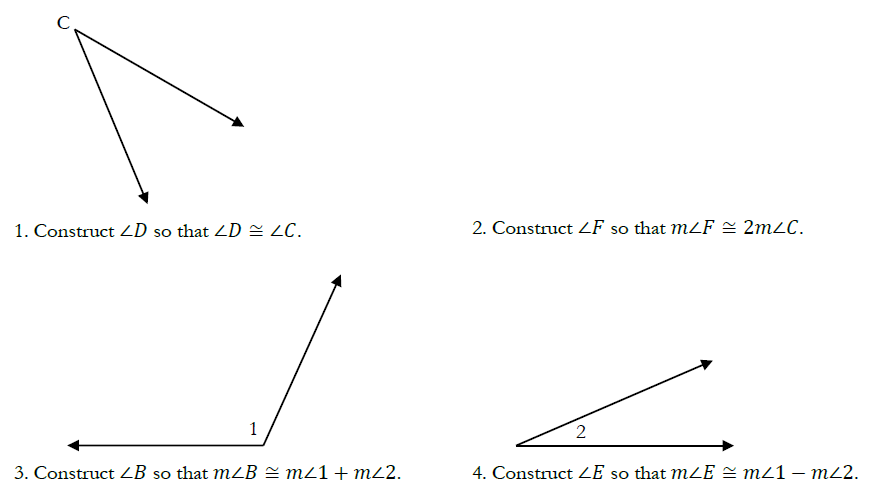Figure 2. Angle Constructions

As with the line segment constructions, students are prompted to share their thinking and reasoning, critique the thinking of others (including the teacher’s), and use appropriate terminology throughout the angle constructions. Students again typically extend the activity by formulating their own problems for one another such as:

• “Describe how to construct an angle H so that the measure of angle H is congruent to the difference of the measure of angle 1 and the measure of angle C”; that is, describe how to construct $$\angle H$$ so that $$m\angle H \cong m\angle 1 - m\angle C$$.

• “Describe how to construct an angle G so that the sum of the measure of angle G and the measure of angle C is congruent to the measure of angle 1”; that is, describe the construction of $$\angle G$$ so that $$m\angle G + m\angle C \cong m\angle 1$$.

As with the student-formulated line segment construction questions, the second author always allows time for students to complete some of these angle construction extension questions. Furthermore, as with the difference of line segments problem—where the second line segment (subtrahend) is longer than the first line segment (minuend)—students frequently question whether the construction $$m\angle 2 - m\angle 1$$ is possible. The second author provides students with time to address this question and promotes the idea that the difference is given by $$\left| m\angle 2 - m\angle 1 \right|$$ (Larson and Boswell, 2019a, p. 39). However, the second author also asserts (to her students) that although the definition of angle measurement does not support the concept of a negative angle, it is frequently useful (e.g., Trigonometry) to impose a convention so that the measure of an “angles is positive when the rotation of its terminal side is counterclockwise and negative when the rotation is clockwise” (Larson and Boswell, 2019b, p. 470).

One of the last constructions that students are asked to create during the second 45-minute class period states:

Construct the perpendicular bisector of $$\overline{\text{XY}}$$ [a given segment]. Then bisect one of the right angles. Measure it with your protractor to check that it is a 45° angle and that your point of intersection divides $$\overline{\text{XY}\ }$$ into two congruent segments.

This problem requires that students incorporate two major concepts of construction: perpendicular and angle bisector. Class discussions related to congruent segments and 45° angles reinforces the definitions of midpoints, angle and segment bisectors, and perpendicular bisectors. Such engagement using hands-on tools and reflective discourse promotes student development of key understandings in geometry and supports geometric reasoning. After completing this last problem, the second author returns to the angle construction activity (Figure 2), where she poses the following questions:

• “Describe how the construction of $$\angle F$$ so that m$$\angle F \cong 2m\angle C\mathrm{\ }$$is related to showing $$\angle C\$$is the angle bisector of $$\angle F$$.”

• “Determine n so that $$n \bullet m\angle C \cong m\angle 1$$.”

This last question involves students making conjectures and attempting to determine n using straightedge and compass via trial and error and inductive reasoning, where, by inductive reasoning, we mean reasoning which places “emphasis on discovering patterns, interpreting information to make generalisations, and making analyses by finding similarities, differences and relations between the attributes” (Misrom et al., 2020, p. 159). This last question further provides students with out-of-class work, as the second author requests that students attempt to solve the problem in pairs or in groups of three.

# CULMINATING CONSTRUCTION ACTIVITY

When finished with the first two 45-minute class periods (i.e., the first two activities), the second author gives her students an assignment that requires them to figure out how to replicate given constructions, such as an eight-petaled rose (based on an octagon) and a 12-pointed flower. Students are required to make their constructions larger than the given diagrams, making it impossible to simply copy the originals. Students use their straightedge and compass along with the understandings and skills they developed in prior lessons and the current sequence of activities to create these new objects. Finally, students are encouraged to color their constructions to enhance their aesthetic beauty.

There are no instructions provided for how to make their construction; rather, students are asked to problem solve and use inductive reasoning in order to create aesthetically pleasing constructions as illustrated in Figure 3. To determine whether an object or item is “aesthetically pleasing,” students are asked to focus on critical features that contribute to aesthetic pleasure, such as “symmetry, balance, proportion, complexity, and so on” (Blijlevens et al., 2014, p. 101). Sample student work is shown in Figure 3.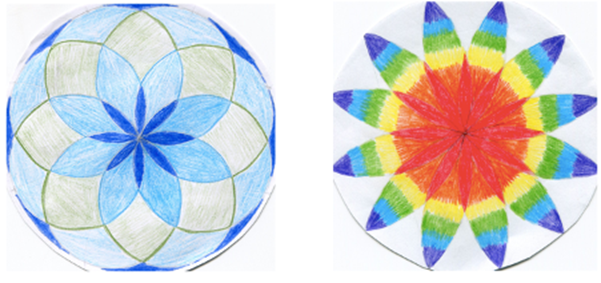Figure 3. Eight-Petaled Rose (left image) and 12-Pointed Flower (right image) Constructions

The eight-petaled rose is created by first constructing an octagon with straightedge and compass. Once students construct the eight points of the octagon, they use inductive reasoning to adjust their compass setting to obtain the small, medium, and large eight-petaled roses. For the 12-petaled flower construction, students first construct the six-petaled flower using their compass and the radius of a circle to create six evenly spaced arcs and points around the circle. Next, students continue using their compass and the same radius to construct six additional evenly spaced arcs and points around the circle. This results in the 12-petaled flower.

Near the end of the third day, the second author takes pictures of each construction and uploads these images to her students’ Chromebooks. Once each student has the image of their construction uploaded to their Chromebook, students use their constructions to decorate the classroom with beautiful artwork (see Figure 4).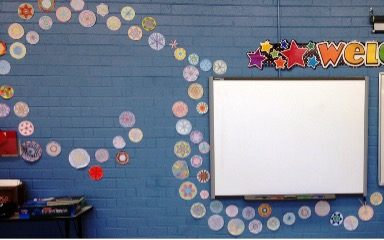Figure 4. Geometric Artwork

Hereafter, this artwork serves as a focus of discourse during parent-teacher conferences and subsequent classes.

# ENHANCING STUDENTS’ GEOMETRIC REASONING

During the fourth and last day of the lesson, the second author integrates GeoGebra’s geometry application into the lesson. GeoGebra is a free interactive geometry, algebra, statistics and calculus application, available on multiple platforms and in over 25 languages, and a tool the second author’s students regularly utilize throughout the school year. Once students have inserted their image into GeoGebra’s interactive geometry environment using GeoGebra’s image tool, the second author discusses selected elements of art, principles of design, and relevant vocabulary to help her students describe and analyze their constructions. Specifically, students are asked to think about line, shape, form, and color, and examine balance (i.e., symmetrical, asymmetrical, radial), pattern, and proportion in their constructions. As described by Esaak (2020), artists may signify a pattern using color, by “repeating a single or select palette of colors throughout a work” (para. 6), or by using lines, shapes, tones, textures and forms (MacTaggert, n.d.). Symmetry (or symmetrical balance) means that “the work of art is the same on one side as the other, a mirror image of itself, on both sides of a center line” (Issaquah Schools Foundation, 2021, para. 2). Conversely, asymmetry (or asymmetrical balance) means that “the two halves of the work of art are different, however . . . there will be elements that interact in a way that makes each side equally important” (Issaquah Schools Foundation, 2021, para. 3). Finally, radial symmetry means “the weight of the image or form radiates from a center point” (Issaquah Schools Foundation, 2021, para. 3).

The final activity involves students developing and analyzing hypotheses regarding their individual constructions, the constructions of two of their classmates, and sharing and discussing their results. Such actions support the integration of geometric constructions, digital technology, elements of art, and principles of design to enhance students’ geometric reasoning. For example, one of the student’s constructions, illustrated in Figure 5, is analyzed using several GeoGebra tools (e.g., point, segment, perpendicular line, and angle measure).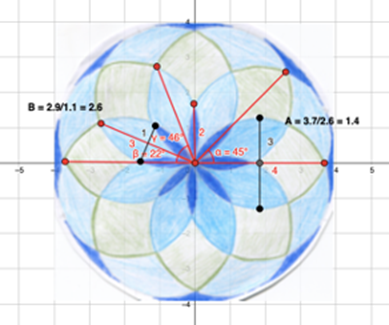Figure 5. Examining the Geometric-Artistic Constructions in GeoGebra

The seven elements of art (i.e., color, form, line, shape, space, texture, and value) are the “building blocks used by artists to create a work of art” (J. Paul Getty Museum, 2011a, p. 1). According to the second author, students typically describe and discuss the lines in their own and their classmates’ constructions by focusing on the existence and placement of vertical, horizontal, diagonal, straight, and curved lines. Students also discuss form and whether an object has width. Finally, students typically focus on each object’s colors including the name of the color used (i.e., hue), and how light or dark the colors are (i.e., value). Sample student responses include:

• “The contrast between the light and dark blues and the grey really make the smallest eight-petaled flower pop out in the drawing” (image on left in Figure 3).

• “I really like how the width of the grey overlapping petals is larger than the width of the light blue petals, which, in turn, is larger than the width of the dark blue petals” (image on left in Figure 3).

• “I wish the contrast between the red flower and the orange circle was greater, because it was challenging for me to see the inner flower” (image on right in Figure 3).

According to the J. Paul Getty Museum (2011b), the principles of design “describe the ways that artists use the elements of art in a work of art” (p. 2) and are comprised of balance, emphasis, movement, pattern, repetition, proportion, rhythm, unity, and variety. According to the second author, students typically discuss ideas of balance, including whether an object has symmetry or radial symmetry. Sample student responses include:

• “The color contrast between the red, orange, and yellow really makes the figure radiate outward from the center of the drawing” (image on right in Figure 3).

• “I think the repeating dark blue-light blue-green-yellow pattern the figure displays on each outer petal is very calming” (image on right in Figure 3).

• “I really thought the proportion of petal length to petal width between the grey petals and the light blue petals was going to be a whole number, like the light blue petal [i.e., petal length to width = 2.9/1.1 = 2.6] proportion was going to be twice as large as the grey petal [i.e., petal length to width = 3.7/2.6 = 1.4] proportion” (Figure 5).

• “Although GeoGebra’s measurement tool indicated the small, dark blue petals occurred every 45°, which is what I expected, the light blue petals did not follow the same pattern, occurring every 44° or 46°. I must have been off somewhere in my construction” (Figure 5).

Though challenging, students find this sequence of activities to be very interesting and enjoyable. Through their engagement in the sequence of activities, students develop a sense of satisfaction and an understanding of the connections between geometry and art.

# DISCUSSION AND CONCLUSION

The work that students do with geometric tools (such as compass and straightedge) helps them develop an understanding of significant geometric connections. Such connections, in turn, supports students’ capacities to prove and problem solve. When students learn a geometric concept that is not coherent, that is, connected to nothing but a theorem in a book, sentences in their notes, or statements made by their teacher, they are not able to provide meaning to that theorem. In the preceding sections, we described a sequence of activities that integrated geometric constructions, digital technology, elements of art, and principles of design to enhance students’ geometric reasoning. More specifically, we demonstrated how students analyzed characteristics and properties of two-dimensional geometric shapes, developed mathematical arguments about geometric relationships, used symmetry to analyze mathematical situations, and used visualization to solve problems.

Several authors have developed and utilized such tools or rubrics (e.g., Isaacson, 2012; Loong et al., 2018; Pegg et al., 1998) and these existing rubrics served as a starting point in our discussions for determining how best to capture and measure these notions. Ayuningtyas et al. (2019) developed assessment criteria for geometric reasoning based on five indicators (p. 4): observe pattern or regularity; formulate generalization and conjecture related with regularity observed; assess/test the conjecture; construct and assess mathematical argument; and describe/validate logical conclusion about some ideas and its relatedness. Burger (2013) developed a series of rubrics for use as part of a project in college-level Geometry that focuses on five criteria: accuracy, neatness, creativity, effort, and meeting deadlines/fallowing directions. Finally, Rajdl (2014) designed a five-point art rubric that incorporates the elements of art and principles of design. We are currently working to integrate these three rubrics into one instrument that would allow teachers to practicably capture and assess students’ capacity to reason in geometry, accurately construction objects with straightedge and compass, and understand and incorporate elements of art and principles of design.

In addition to the lack of an instrument to measure students’ understandings and skills, study limitations also included the small student sample, and the lack of video recordings or transcriptions of classroom discussions. The National Council for Teachers of Mathematics has long encouraged math teachers to “develop multiple representations and connections, and construct meanings from new situations” (NCTM, 1989, p. 125). The sequence of activities described in this article, implemented through a STEAM approach that focuses on the benefits of integrated learning (Liao, 2019), provide students with opportunities to develop such meanings and connections in the context of creating artistic geometric constructions.Figure 1. Line Segment ConstructionsFigure 2. Angle ConstructionsFigure 3. Eight-Petaled Rose (left image) and 12-Pointed Flower (right image) ConstructionsFigure 4. Geometric ArtworkFigure 5. Examining the Geometric-Artistic Constructions in GeoGebra
AMA 10th edition
In-text citation: (1), (2), (3), etc.
Reference: Courtney SA, Armstrong B. Promoting Geometric Reasoning through Artistic Constructions. European Journal of STEM Education. 2021;6(1), 09. https://doi.org/10.20897/ejsteme/11332
APA 6th edition
In-text citation: (Courtney & Armstrong, 2021)
Reference: Courtney, S. A., & Armstrong, B. (2021). Promoting Geometric Reasoning through Artistic Constructions. European Journal of STEM Education, 6(1), 09. https://doi.org/10.20897/ejsteme/11332
Chicago
In-text citation: (Courtney and Armstrong, 2021)
Reference: Courtney, Scott A., and Brittany Armstrong. "Promoting Geometric Reasoning through Artistic Constructions". European Journal of STEM Education 2021 6 no. 1 (2021): 09. https://doi.org/10.20897/ejsteme/11332
Harvard
In-text citation: (Courtney and Armstrong, 2021)
Reference: Courtney, S. A., and Armstrong, B. (2021). Promoting Geometric Reasoning through Artistic Constructions. European Journal of STEM Education, 6(1), 09. https://doi.org/10.20897/ejsteme/11332
MLA
In-text citation: (Courtney and Armstrong, 2021)
Reference: Courtney, Scott A. et al. "Promoting Geometric Reasoning through Artistic Constructions". European Journal of STEM Education, vol. 6, no. 1, 2021, 09. https://doi.org/10.20897/ejsteme/11332
Vancouver
In-text citation: (1), (2), (3), etc.
Reference: Courtney SA, Armstrong B. Promoting Geometric Reasoning through Artistic Constructions. European Journal of STEM Education. 2021;6(1):09. https://doi.org/10.20897/ejsteme/11332